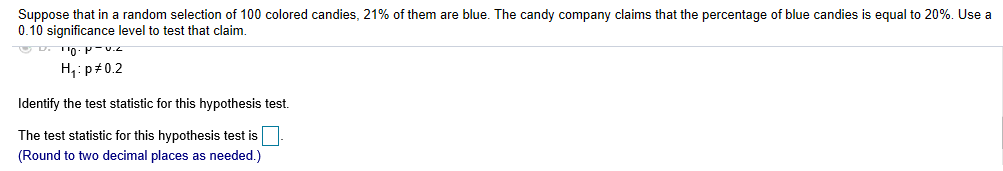# Suppose that in a random selection of 100 colored candies, 21% of them are blue. The candy company claims that the percentage of blue candies is equal to 20%. Use a 0.10 significance level to test that claim. H, p#0.2 Identify the test statistic for this hypothesis test. The test statistic for this hypothesis test is (Round to two decimal places as needed.)

Question
16029 viewshelp_outlineImage TranscriptioncloseSuppose that in a random selection of 100 colored candies, 21% of them are blue. The candy company claims that the percentage of blue candies is equal to 20%. Use a 0.10 significance level to test that claim. H, p#0.2 Identify the test statistic for this hypothesis test. The test statistic for this hypothesis test is (Round to two decimal places as needed.) fullscreen

### Want to see the step-by-step answer?